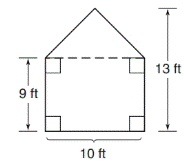Chapter 8.1, Problem 16EElementary Geometry For College St...

7th Edition
Alexander + 2 others
ISBN: 9781337614085

Solutions

Chapter
SectionElementary Geometry For College St...

7th Edition
Alexander + 2 others
ISBN: 9781337614085
Textbook Problem

In Exercises 9 to 18, find the areas of the figures shown or described.To determine

To find:

The area of given figure.

Explanation

1) Formula:

Finding the area of the figure is followed by identifying the figure and find area with the suitable geometry formulae,

Area of rectangle A=lw unit2

Where, l is the length of rectangle and w is the width of rectangle.

Area of triangle A=12bh unit2

Where, b is the base of triangle and h is the height of triangle.

2) Calculation:

Given,

This is the compound figure of rectangle and triangle.

Area of given figure(A)=Area of rectangle(A1)+Area of a triangle(A2)

Area of rectangle:

A1=lw unit2

Where, l is the length of rectangle 10 ft

w is the width of rectangle 9 ft

A1=10(9)

A1=90 ft2

Area of triangle:

A2=12bh unit2

Still sussing out bartleby?

Check out a sample textbook solution.

See a sample solution

The Solution to Your Study Problems

Bartleby provides explanations to thousands of textbook problems written by our experts, many with advanced degrees!

Get Started

Evaluate the integral, if it exists. exxdx

Single Variable Calculus: Early Transcendentals, Volume I

Find all possible real solutions of each equation in Exercises 3144. y3+64=0

Finite Mathematics and Applied Calculus (MindTap Course List)

What are the two requirements for a random sample?

Statistics for The Behavioral Sciences (MindTap Course List)